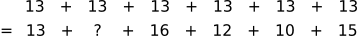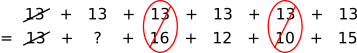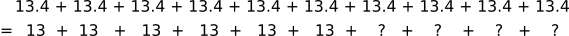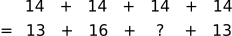#### You may also like### Kate's Date

When Kate ate a giant date, the average weight of the dates decreased. What was the weight of the date that Kate ate?### Balancing the Books

How many visitors does a tourist attraction need next week in order to break even?### Grandpa's Rock Cakes

Grandpa made a super-heavy rock cake. Five people have guessed the weight of the cake. Can you work out the weight of his cake?

# Bat Wings

##### Age 11 to 14 ShortChallenge Level

Working backwards
Student A's bats have an average wingspan of $13$ cm. Student A has $6$ bats, and so the sum of their wingspans divided by $6$ is equal to $13$.

So the sum of student A's bats' wingspans is $6\times13=78$

So the missing measurement can be found by subtracting the five known wingspans from the total: $78 - (13+16+12+10+15) = 12$ cm.

Next, we can find student B's mean using the total mean:
There are $10$ bats altogether, and the average wingspan is $13.4$ cm, so the total wingspan is $10\times13.4 = 134$ cm.

We already know that student A's bats' had a total wingspan of $78$ cm. So student B's bats' total wingspan must be $134 - 78 = 56$ cm.

So B's missing measurement can be found by subtracting the three known wingspans from the total: $56 - (13+16+13) = 14$ cm.

By 'balancing' the numbers
We can think of the average bat wingspan as being the wingspan that each bat would get if the total amount of wing were shared out equally between all of the bats.

So total wingspan of student A's bats is the same as the total wingspan of 6 bats which each had a wingspan of 13 cm. We can write them below each other and then make the sums 'balance':Notice that the first 13 has 13 below it, so these are already balanced. 16 is 3 more than 13 and 10 is 3 less than 13, so together 10 and 16 balance out 13 and 13. This is shown below:12 and 15 do not balance with 13 and 13, because 12 is 1 less than 13 but 15 is 2 more than 13. That is 1 below but 2 above, so we need another 1 below in order to balance the sums.

So the missing measurement must be 12.

Then, we can find the average for student B.
Altogether there are 10 bats, and if the wingspan was shared out equally, each would get 13.4 cm. Alternatively, we could share the wingspan out by student. Student A's bats would each get 13 cm and student B's bats would each get ? cm (their respective averages). This is shown below:But student A's bats each get 0.4 less than 13.4, so in total, they contribute 6$\times$0.4 less than their fair share to the total. So student B's bats must contribute 6$\times$0.4 more than their fair share to the sum.

6$\times$0.4 is the same as 4$\times$0.6, so each of student B's 4 bats could have 0.6 cm more than the average wingspan - which is 13.6 + 0.6 = 14 cm.

Finally, we can find student B's missing measurement:The 13s are each 1 below 14, and the 16 is 2 above 14. So in the bottom sum, we have 2 below and 2 above 14 - which balances out to 14! So the sums are already balanced, so ? must be 14 to maintain this balance.

So student B's missing measurement is 14 cm.

You can find more short problems, arranged by curriculum topic, in our short problems collection.